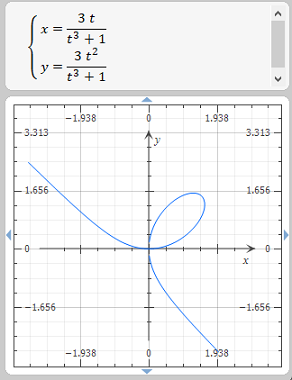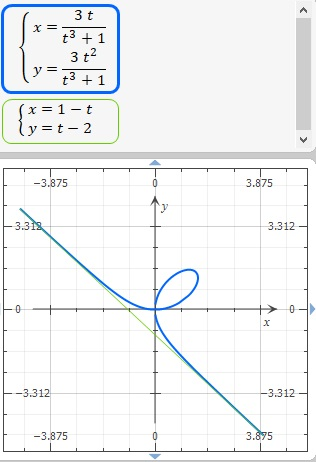# 1. A curve called folium of Descartes is defined by the parametric equation x= \frac{3t}{1+t^3},...

## Question:

1. A curve called folium of Descartes is defined by the parametric equation {eq}x= \frac{3t}{1+t^3}, y= \frac{3t^2}{1+t^3} {/eq}

Show that if the point {eq}(a,b){/eq} lies on the curve so does the point {eq}(b,a){/eq} This shows that the curve is symmetric about the line {eq}y=x{/eq} Where does the curve intersect the line {eq}y=x{/eq}?

2. Find the points on the curve where the tangent lines are horizontal or vertical.

a line {eq}y=mx+b{/eq} is called a slant assymptote of the curve {eq}y=f(x){/eq} of {eq}\lim_{x \rightarrow \infty} (f(x)-mx+b)=0{/eq} Show that {eq}y=-x-1{/eq} is a slant adsymptote of the curve

## Asymptotes and Tangent Lines to a Parametric Curve:

A parametric curve is expressed using parametric equations of the form,

{eq}x = x(t) \\ y = y(t) {/eq}

The slope of the curve is then computed as,

{eq}\displaystyle \frac{dy}{dx} = \frac{\frac{dy}{dt}}{\frac{dx}{dt}} {/eq}

The above expression gives us the slope of the tangent to the curve, and the equation of the tangent can be found easily once we know the slope, using the equation of a line with known slope {eq}m {/eq} and a point {eq}(x_0, y_0) {/eq} through which the line passes as,

{eq}y - y_0 = m ( x - x_0) {/eq}

Asymptotes are lines to which the graph of the function becomes parallel to as {eq}x \to \infty {/eq} or {eq}y \to \infty {/eq}.

There are horizontal, vertical and oblique asymptotes possible for a curve.

1. We are given the curve called folium of Descartes defined by the parametric equation

{eq}\displaystyle x= \frac{3t}{1+t^3}, \; y= \frac{3t^2}{1+t^3} {/eq}

Observe the graph of the curve called Folium of Descartes:a) Show that if the point {eq}(a,b){/eq} lies on the curve so does the point {eq}(b,a){/eq} This shows that the curve is symmetric about the line {eq}y=x{/eq}

In other words we must show that the x and y coordinate can be interchanged and the equation of the curve should remain the same.

From the parametric equations, we have,

{eq}\begin{align*} x &= \frac{3t}{1+t^3} \\ x^3 &= \frac{27t^3}{(1+t^3)^3} \\ y &= \frac{3t^2}{1+t^3} \\ y^3 &= \frac{27t^6}{(1+t^3)^3} \\ x^3 + y^3 &= \frac{27t^3( 1 + t^3)}{(1+t^3)^3} \\ &= \frac{27t^3}{(1+t^3)^2} \\ &= 3xy \\ x^3 + y^3 &= 3 xy \end{align*} {/eq}

We have arrived at the Cartesian equation of the curve which shows that interchanging the x and y coordinate does not change the equation itself. Hence we conclude that if the point {eq}(a,b) {/eq} lies on the curve, the point {eq}(b,a) {/eq} must also lie on the curve. Note that It is also evident from the graph of the curve that the Folium of Descartes is symmetric about the line {eq}y = x. {/eq}.

b) Where does the curve intersect the line {eq}y=x{/eq}?

Where the curve intersects line {eq}y = x {/eq} the parametric coordinates of the curve must be equal. From the parametric equations we get,

{eq}\begin{align*} x &= \frac{3t}{1+t^3} \\ y &= \frac{3t^2}{1+t^3} \\ \frac{3t^2}{1+t^3} &= \frac{3t}{1+t^3} \\ t &= 0, \; t = 1 \end{align*} {/eq}

The curve intersects the line {eq}y = x {/eq} at the points, {eq}t = 0, \; t = 1 {/eq}. We assume the denominator is not zero, i.e. {eq}t^3 +1 \neq 0 \leftrightarrow t \neq -1 {/eq}.

2. a) Find the points on the curve where the tangent lines are horizontal or vertical.

The curve has a horizontal tangent where,

{eq}\frac{dy}{dt} = 0, \; \text{and} \frac{dx}{dt} \neq 0 {/eq}

We compute the first order derivatives from the parametric equations:

{eq}\begin{align*} y &= \frac{3t^2}{1+t^3} \\ \frac{dy}{dt} &= \frac{( 1 + t^3) 6t -3t^2(3t^2)}{(1 + t^3)^2} \\ &= \frac{6t + 6t^4 - 9t^4}{(1 + t^3)^2} \\ &= \frac{6t - 3t^4}{(1 + t^3)^2} \\ &= 3t \times \frac{(2 - t^3)}{(1 + t^3)^2} \\ x &= \frac{3t}{1+t^3} \\ \frac{dx}{dt} &= \frac{( 1 + t^3)3 - 3t( 3t^2)}{(1 + t^3)^2} \\ &= \frac{3( 1 + t^3 - 3t^3)}{(1 + t^3)^2} \\ &= \frac{3( 1 - 2 t^3)}{(1 + t^3)^2} \\ \end{align*} {/eq}

It is evident that there is a horizontal asymptote when,

{eq}\frac{dy}{dt} = 0 {/eq}.

when {eq}t = 0 {/eq} which implies, {eq}x = y = 0 {/eq} which is at the origin.

The other point where {eq}\frac{dy}{dt} = 0 {/eq} is at,

{eq}2 - t^3 = 0 \Longrightarrow t^3 = 2 \Longrightarrow t = ( 2)^{1/3} {/eq}

The vertical asymptote is at the point where,

{eq}\frac{dx}{dt} = 0 \Longrightarrow 1 - 2 t^3 = 0 \Longrightarrow t^3 = \frac{1}{2} \Longrightarrow t = (\frac{1}{2} )^{1/3} {/eq}

b). A line {eq}y=mx+b{/eq} is called a slant assymptote of the curve {eq}y=f(x){/eq} of {eq}\lim_{x \rightarrow \infty} (f(x)-mx+b)=0{/eq} Show that {eq}y=-x-1{/eq} is a slant asymptote of the curve

We know that {eq}y = f(x) \to mx + b {/eq} when {eq}x \to \infty {/eq}.

We substitute this value for {eq}y {/eq} in the cartesian equation of the curve and try to solve for {eq}m, \; b {/eq} as follows:

{eq}\begin{align*} x^3 + y^3 &= 3xy \\ x^3 + ( mx + b)^3 &= 3x ( mx + b) \\ x^3 + m^3x^3 + 3m^2x^2b + 3mxb^2 + b^3 &= 3mx^2 + 3b x\\ x^3( 1 + m^3) + x^2( 3m^2b - 3m) + x(3mb^2 - 3b) &= 0 \\ \end{align*} {/eq}

Setting the like coefficients to zero in the above equation we get,

{eq}1 + m^3 = 0 \Longrightarrow m^3 = -1 \Longrightarrow m = -1 \\ 3m^2b - 3m = 0 \Longrightarrow 3m( mb -1) = 0 \Longrightarrow mb - 1 = 0 \Longrightarrow b = -\frac{1}{m} = -1 \\ 3mb^2 - 3b = 0 \Longrightarrow mb = 1 \Longrightarrow b = \frac{1}{m} = -1 {/eq}

{eq}\text{Therefore we have proved that } y = -x -1 \text{ is an asymptote to the given curve.} {/eq}

Here is an alternate way to show that the given line is an asymptote. The slope of the line {eq}y = -x -1 {/eq} is, {eq}m = -1 {/eq}.

The slope of the given curve is given by,

{eq}\begin{align*} \frac{dy}{dt} &= 3t \times \frac{(2 - t^3)}{(1 + t^3)^2} \\ \frac{dx}{dt} &= \frac{3( 1 - 2 t^3)}{(1 + t^3)^2} \\ \frac{dy}{dx} &= \frac{3t ( 2 -t^3)}{3( 1 - 2t^3)} \\ \end{align*} {/eq}

When {eq}\displaystyle x = \frac{3t}{1+t^3} \to \infty \Longrightarrow t^3 = -1 \Longrightarrow t = -1 {/eq}

Substituting this value in the derivative to find the slope of the curve when {eq}x \to \infty {/eq} we get,

{eq}\begin{align*} \frac{dy}{dx}\bigg|_{x \to \infty} &= \frac{dy}{dx}\bigg|_{t \to -1} \\ &= \frac{3(-1) ( 2 - (-1)^3)}{3( 1 - 2(-1)^3}\\ &= \frac{-1( 2 + 1)}{1-2(-1)}\\ &= \frac{-1( 3)}{3}\\ &= - 1 \end{align*} {/eq}

The slope of the curve matches the slope of the given line as {eq}x \to \infty {/eq} hence we conclude the given line must be a slant asymptote of the given function. This is true as shown by the following graph:The graph of the line {eq}x + y + 1 = 0 {/eq} is also shown using its parametric form to match the parametric equations of the curve.